Exhibit 99.1How AI + Collaboration Transformed Professional Learning A P R I L 4 , 2 0 2 2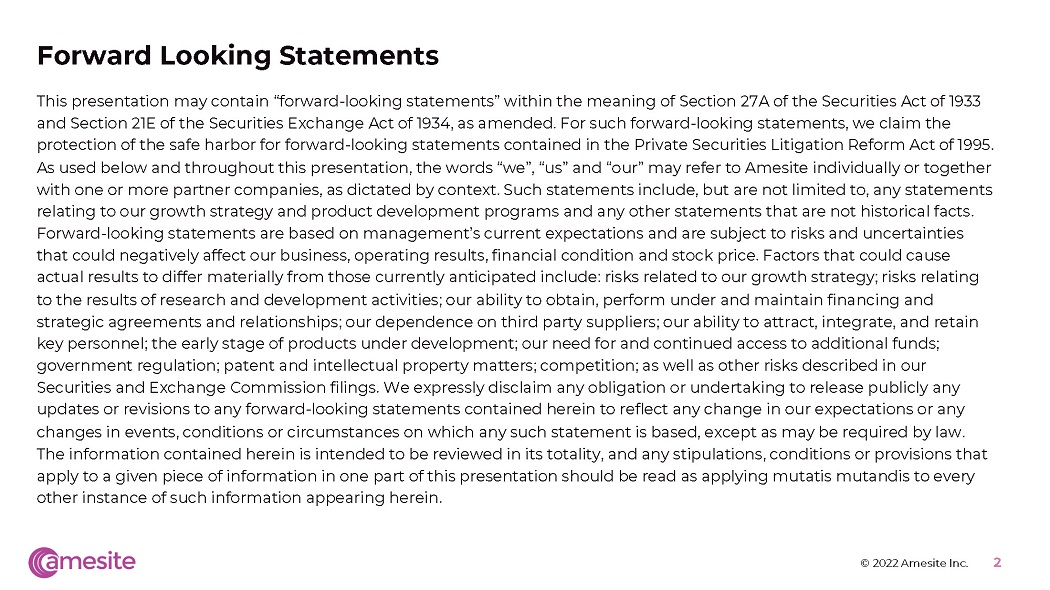Forw a rd L ooki ng S t a t e m e n t s This presentation may contain “forward - looking statements” within the meaning of Section 27A of the Securities Act of 1933 and Section 21E of the Securities Exchange Act of 1934, as amended. For such forward - looking statements, we claim the protection of the safe harbor for forward - looking statements contained in the Private Securities Litigation Reform Act of 1995. As used below and throughout this presentation, the words “we”, “us” and “our” may refer to Amesite individually or together with one or more partner companies, as dictated by context. Such statements include, but are not limited to, any statements relating to our growth strategy and product development programs and any other statements that are not historical facts. Forward - looking statements are based on management’s current expectations and are subject to risks and uncertainties that could negatively affect our business, operating results, financial condition and stock price. Factors that could cause actual results to differ materially from those currently anticipated include: risks related to our growth strategy; risks relating to the results of research and development activities; our ability to obtain, perform under and maintain financing and strategic agreements and relationships; our dependence on third party suppliers; our ability to attract, integrate, and retain key personnel; the early stage of products under development; our need for and continued access to additional funds; government regulation; patent and intellectual property matters; competition; as well as other risks described in our Securities and Exchange Commission filings. We expressly disclaim any obligation or undertaking to release publicly any updates or revisions to any forward - looking statements contained herein to reflect any change in our expectations or any changes in events, conditions or circumstances on which any such statement is based, except as may be required by law. The information contained herein is intended to be reviewed in its totality, and any stipulations, conditions or provisions that apply to a given piece of information in one part of this presentation should be read as applying mutatis mutandis to every other instance of such information appearing herein. © 2022 Amesite Inc. 2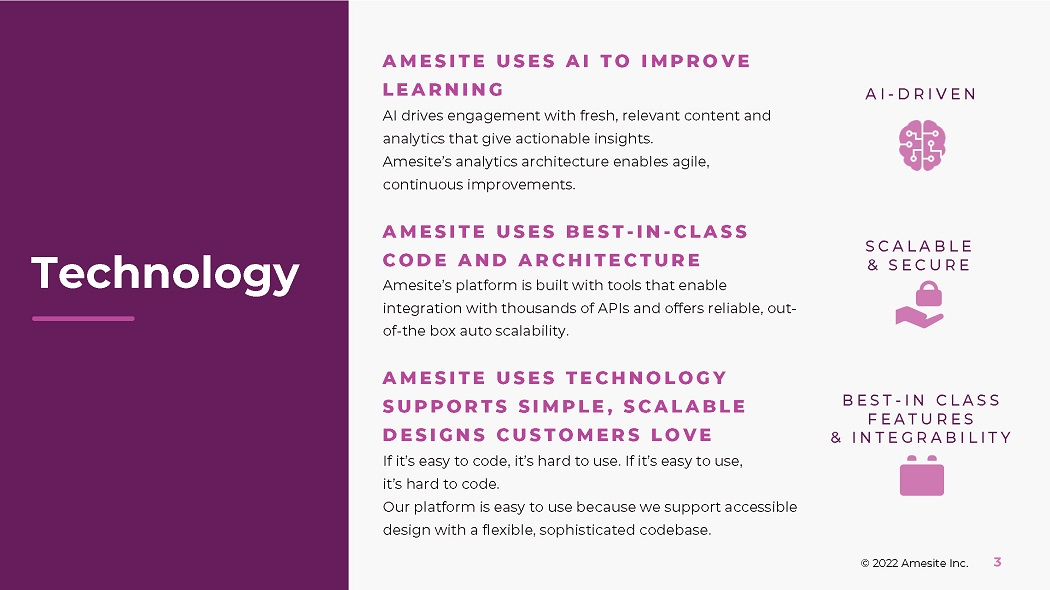T e c hn o l o gy S C A L A B L E & S E C U R E A I - D R I V E N B E S T - I N C L A S S F E A T U R E S & I N T E G R A B I L I T Y A M E S I T E U S E S A I T O I M P R O V E L E A R N I N G © 2022 Amesite Inc. 3 AI drives engagement with fresh, relevant content and anal yt i c s t ha t g i v e a c t i o na b l e i n s i ght s . Amesite’s analytics architecture enables agile, c o n t i nu o u s i m p r o v e m e n t s . A M E S I T E U S E S B E S T - I N - C L A S S C O D E A N D A R C H I T E C T U R E A m e s i te ’ s p l a t f o r m i s b u il t w i t h t oo l s t h a t e n a b l e i n te g r a t io n w it h t h o us a n d s o f A P I s a n d o ff e r s r e li a b le , o u t - of - t h e b o x a u t o s c a l a b ili ty . A M E S I T E U S E S T E C H N O L O G Y S U P P O R T S S I M P L E , S C A L A B L E D E S I G N S C U S T O M E R S L O V E I f i t ’ s e a s y t o c o d e , i t ’ s ha r d t o u s e . I f i t ’ s e a s y t o u s e , it ’ s h a r d t o c o d e . O u r p l a tfo r m i s e a s y t o u s e b e c a u s e w e s u pp o r t a cc e ss i b l e design with a flexible, sophisticated codebase.M a r ke t s i z e o f t h e m u s e u m in d u s t r y i n t h e U S r e a ch e d \$1 2 . 7 B i n 2020 © 2 0 2 2 A m e s i t e I nc . 4 P a r tn e r s hi p s and Impact: >98% retention CITY UNIVERSITY OF NEW YORK • I n f r a s t r u c tu r e t r a i n i n g fo r u n de r s e r v e d l e a r n e rs at the largest urban university system in the United States WAYNE STATE UNIVERSITY • F u t u r e o f M o b ili t y t r a i n i n g t o t h e G r e a t e r D e t r o i t Ar e a a s Mi c h i g a n ’ s t h i r d - l a r g e s t un iv e r s ity MICHIGAN WORKS! SOUTHEAST • F u t u r e o f W o r k t r a i n i n g t o a n d a g e n c y t h a t supports nearly 18,000 community members THE HENRY FORD MUSEUM • I n n ov a ti on f or T e a c h e r s t r a i n i n g t o a n o r g a n i z a ti o n t h a t i s ra p i d l y d i g i t a li z i n g o ff e r i n g s REAGAN PRESIDENTIAL INSTITUTE • Communications training for an organization that is rapidly expanding access INNOVATION INSTITUTE - CATHIE WOOD, CEO • I n n vov a ti on t r a i n i n g fo r t e a c h e r s i n pa r t n e rs h i ps with school districts FORD MOTOR COMPANY • AI t r a i n i n g fo r R&D l e a de r s t o b u il d k n o w - ho w i n A I and AI team formation https://www1.nyc.gov/site/planning/about/press - releases/pr - 20210720.page https:// www.statista.com/statistics/1232500/size - of - the - workplace - training - market - north - america - and - rest - of - the - world / https:// www.statista.com/statistics/738519/workplace - training - spending - per - employee/ https:// www.weforum.org/agenda/2021/02/most - in - demand - jobs - 2021 / https:// www.statista.com/statistics/1174784/museum - industry - market - size - us / U p s k illi n g i s a \$358B global market with em p l o ye r s s p e n d i n g \$1 , 3 0 0 o n e a c h e m p l o y e e a nn u a ll y fo r c o n t i nu i n g e d uc a t i o n 150M n e w t e c hn o l o g y j o b s w i l l b e a d d e d t o t h e g l o b a l e c o n o m y o v e r t h e n ex t 5 y ea r s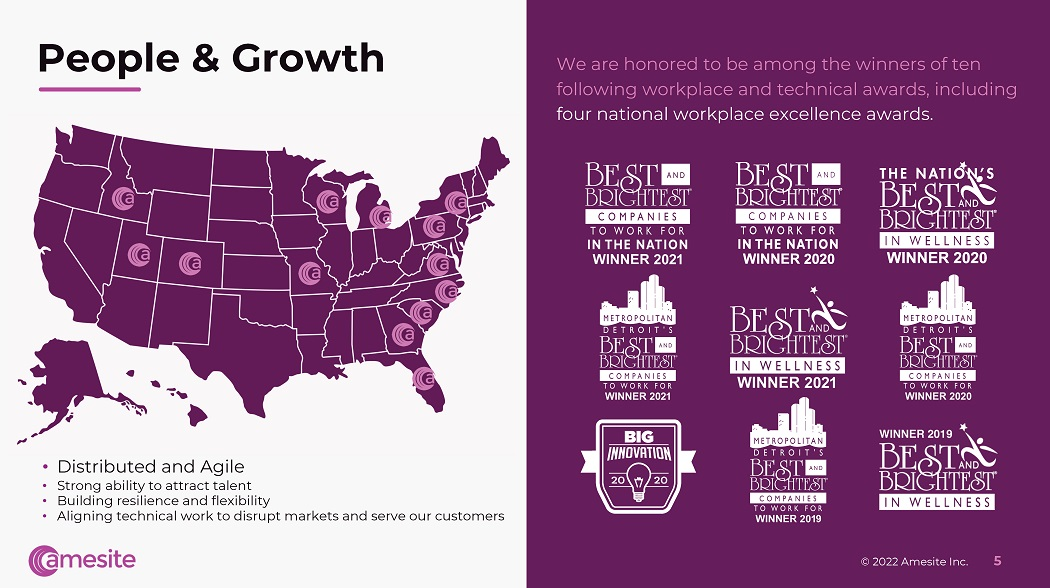People & Growth W e a r e h o n or e d t o b e a m o n g t h e w i nn e r s of t e n fo llo w i n g w o r k p la c e a n d t e c hn ic a l a w a r d s , i n c lu d i n g fo u r n a t io n a l w o r k p la c e e xc e lle n c e a w a r d s . • D i s t r i b u t e d a n d Ag il e • S t r o n g a b ili t y t o a tt r a c t t a l e n t • Bu il d i n g r e s ili e nc e a n d f l e x i b ili t y • Aligning technical work to disrupt markets and serve our customers © 2022 Amesite Inc. 5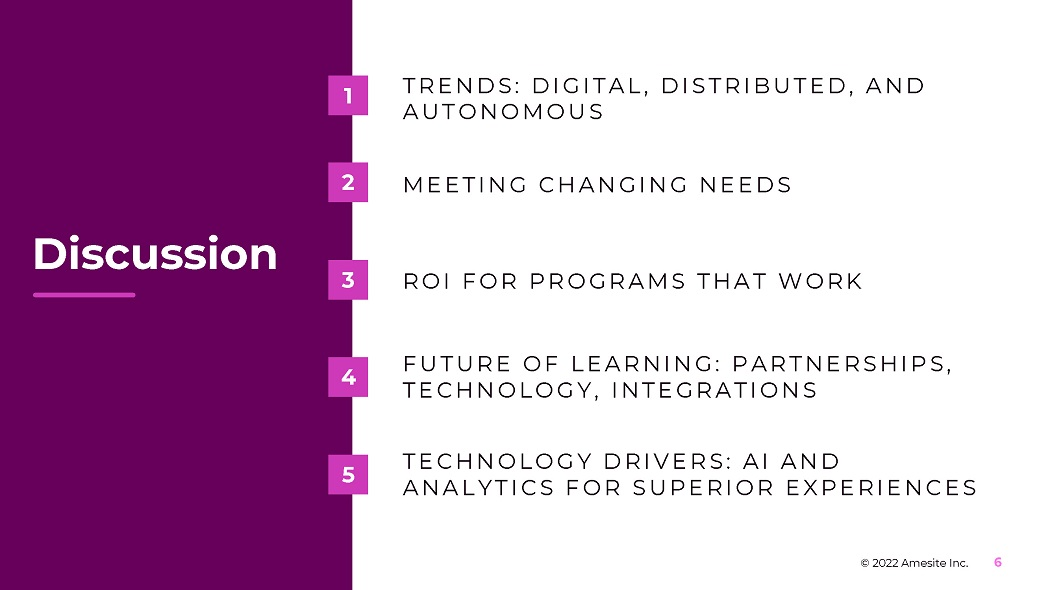Discussion T R E N D S : D I G I T A L , D I S T R I B U T E D , A N D AUT O N O MO US 1 M E E T I N G C H A N G I N G N E E D S 2 R O I F O R P R O GRA M S T H A T W O R K 3 F U T U R E O F L E A R N I N G : P A R T N E R S H I P S , T E C H N O L O G Y , I N T E G R A T I O N S 4 T E C H N O L O G Y D R I V E R S : A I A N D A N A L Y T I C S F O R S U P E R I O R E X P E R I E N C E S 5 © 2022 Amesite Inc. 6T r e n ds © 2 0 2 1 A m e s i t e I nc . A l l R i g h t s R e s e r v e d . 7© 2 0 2 2 A m e s i t e I nc . 8 The Future of Work: More Digital Remote Workers are Happier and Stay in their Jobs Longer % of Employees Working Remote https://amesite.com/blogs/is - remote - work - here - to - stay/ 22% 5 9 % h a pp i e r w o r k i ng a t h o me t h a n w o r k e rs w h o a l w a y s w o r k o n s i te m o r e li k e l y t o c h o o s e a n e mp l o y e r w ho offered remote work compared to those w h o d i d n ’ t. … r e m o te w o r k e r s r e p o r t e d h a v i n g l e s s s t r e ss , m o re f o cu s , a n d a b ette r w o r k - lif e b a l a n c e . ” “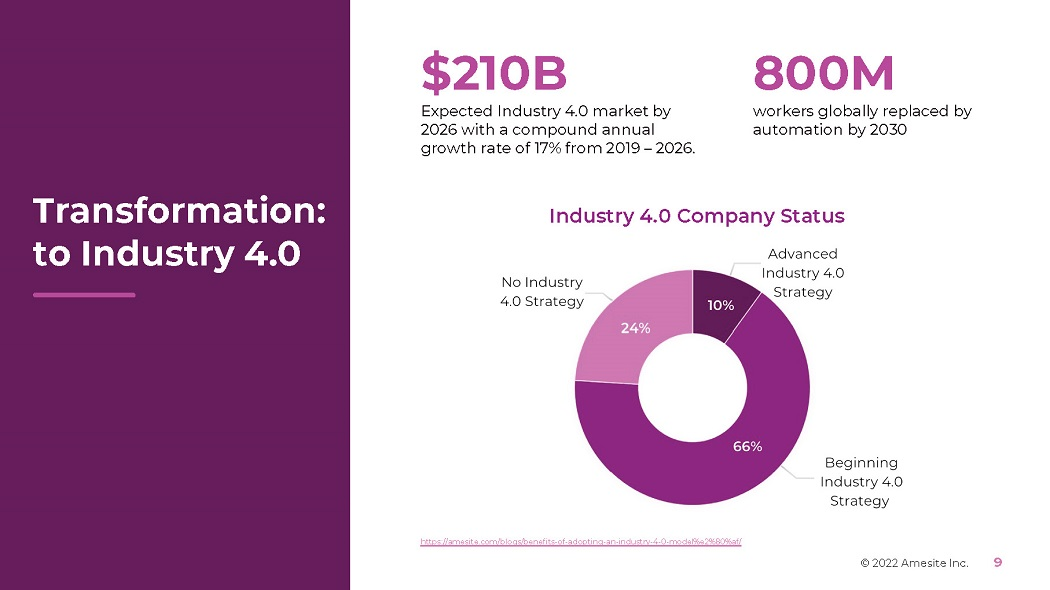T r a n s f o r m a t i o n : to Industry 4.0 \$210B Ex pe c t e d I n d u s t ry 4 . 0 m a r k et by 2 0 26 w i t h a c o m p o u n d a nn u a l gr o w t h r a t e o f 1 7 % f r o m 2 0 1 9 – 2 0 2 6 . Industry 4.0 Company Status 800M w o r k e r s g l o b a ll y r e p l a c e d b y a u t o m a t io n b y 2 0 3 0 © 2022 Amesite Inc. 9 https://amesite.com/blogs/benefits - of - adopting - an - industry - 4 - 0 - model%e2%80%af/The U.S. Bureau of Labor Statistics (BLS) projects there will be 11.9 million new jobs created from 2020 to 2030 , an overall growth rate of 7.7% . © 2022 Amesite Inc. 10 Occupation P er c e n t e m pl o ym e n t change, 2020 – 2030P Num er i c e m pl o ym e n t change, 2020 - 2030P Median ann u a l w a g e , 2020 W i n d t u rb i n e serv i c e t echn i c i an s 68.2% 4,700 \$56,230 N u rs e prac t i t i oner s 52.2% 114,900 \$111,680 Solar photovoltaic installers 52.1% 6,100 \$46,470 Statisticians 35.4% 14,900 \$92,270 P hys i ca l t herap i s t ass i s t an t s 35.4% 33,200 \$59,770 Infor m a t i o n se cu r i t y ana l ys t s 33.3% 47,100 \$103,590 Ho me hea l t h an d persona l car e a i de s 32.6% 1,129,900 \$27,080 Med i c a l an d hea l t h serv i c e s m ana g er s 32.5% 139,600 \$104,280 Da t a s c i en t i s t s an d m a t he m a t i c a l sc i enc e occ u pa t i ons , a l l o t he r 31.4% 19,800 \$98,230 P hys i c i a n ass i s t an t s 31.0% 40,100 \$115,390 T h e T o p 1 0 F a s t e s t G r o w i n g J o b s Market Changes and New Jobs https://amesite.com/blogs/university - presidents - you - can - spearhead - professional - learning - that - drives - revenue/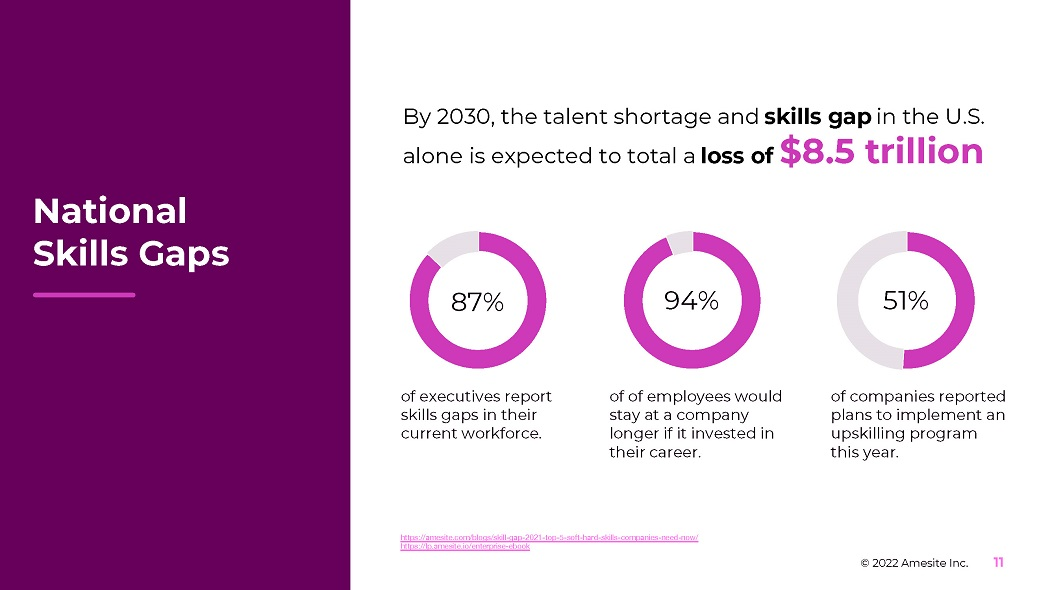of e x e c u t i v e s r e p o r t s k ill s g a p s i n t h e i r cu rr e n t w o r k f o r c e . 8 7 % 94% of of e m p l o y ee s w ou l d s t a y a t a c o m p a n y lo n g e r i f i t i n v e s t e d in t h e i r c a r ee r . 5 1 % © 2022 Amesite Inc. 11 of c o m p a n i e s r e p o r t e d p l a n s t o i m p le m e n t a n u p s k illi n g p r o g r a m t h i s y e a r . https://amesite.com/blogs/skill - gap - 2021 - top - 5 - soft - hard - skills - companies - need - now/ https://lp.amesite.io/enterprise - ebook By 2030, the talent shortage and skills gap in the U.S. alone is expected to total a loss of \$8.5 trillion National Skills Gapsof S t a t e G o v e r n o r s a g r ee t h a t w o r k f o r ce de v e l o pm e n t i s a t o p priority for state and federal efforts. 8 4 % 64% of L & D p r of e ss i o n a l s a g r ee t h a t L & D s h i f t e d fr o m a “ n i c e t o h a v e ” t o a “ n ee d t o h a v e ” i n 2 0 2 1 . 4 7 % of U S j o b s fa c e p o t e n t i a l au t o m a t i o n o v e r t h e n e x t 2 0 y e a r s . US F e d e r a l G o v e r n m e n t a ll o c a t e d o v e r \$3 . 6 B o f bu dge t towards Training and Employment Services in 2021 © 2022 Amesite Inc. 12 Government Recognizes the Skills Gaps https://amesite.com/blogs/a - history - how - government - policy - shaped - the - relationship - between - community - colleges - and - workforce - devel opment/) https://lp.amesite.io/gov - ebook https://amesite.com/blogs/upskilling - employees - for - the - future - of - work / https://amesite.com/blogs/transformation - of - manufacturing - industries /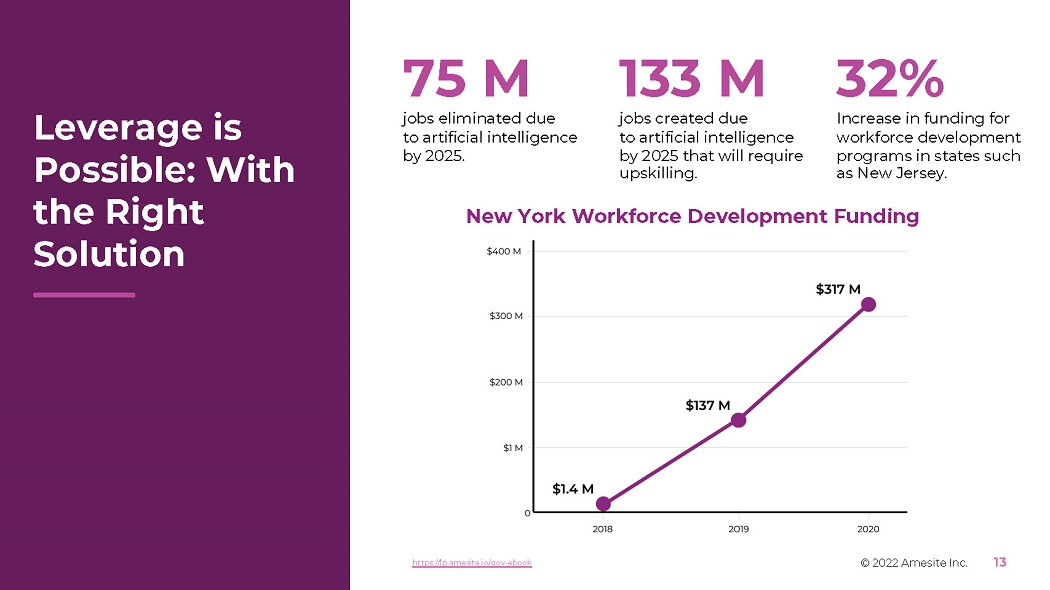© 2 0 2 2 A m e s i t e I nc . 13 N e w Yor k W or k f or c e D e v e lopm e n t F u n d i n g 75 M j o b s e li m i n a t e d d u e t o a r ti f i c i a l i n t e lli g e n c e by 2 0 2 5 . 133 M j o b s c r e a t e d d u e t o a r ti f i c i a l i n t e lli g e n c e by 2 0 2 5 t h a t w il l r e q u ire upskilling. https://lp.amesite.io/gov - ebook 32% In c r e a s e i n f u nd i ng f o r w o r k f o r c e d e v e l o p m e n t pro g r a m s i n s t a t e s s uc h a s N e w J e r s e y . Leverage is Possible: With the Right SolutionMeeting Changing Needs © 2 0 2 1 A m e s i t e I nc . A l l R i g h t s R e s e r v e d . 14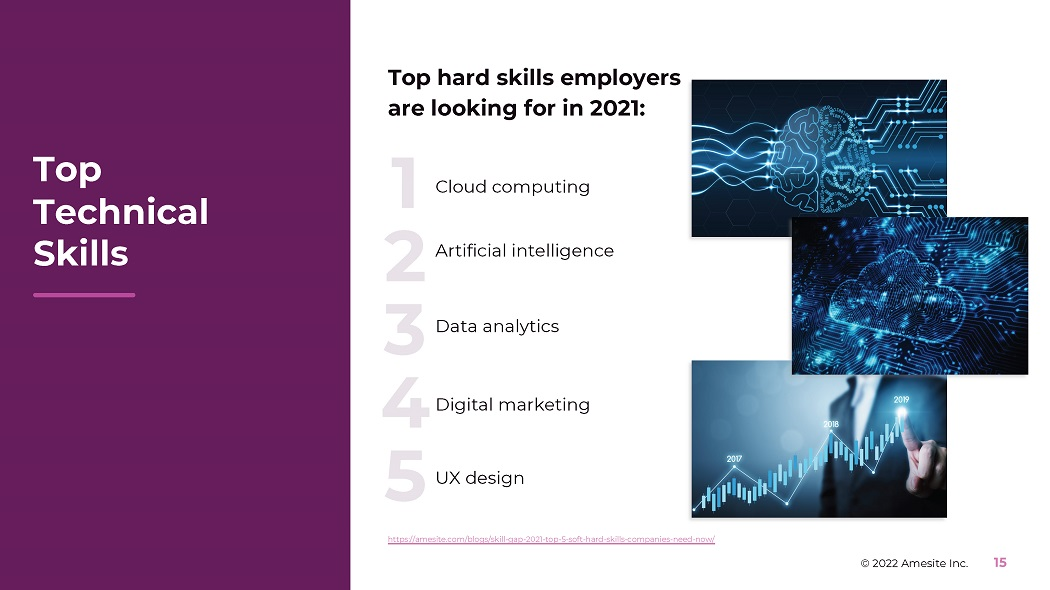C l o u d co m p u t i n g https://amesite.com/blogs/skill - gap - 2021 - top - 5 - soft - hard - skills - companies - need - now/ © 2022 Amesite Inc. 15 1 2 A r t i f i c i a l i n t e lli g e n c e 3 D a t a a n a l y t i c s 4 D i g i t a l m a r k e t i n g 5 UX d e s i g n Top hard skills employers are looking for in 2021: Top Te c h n i c a l Skills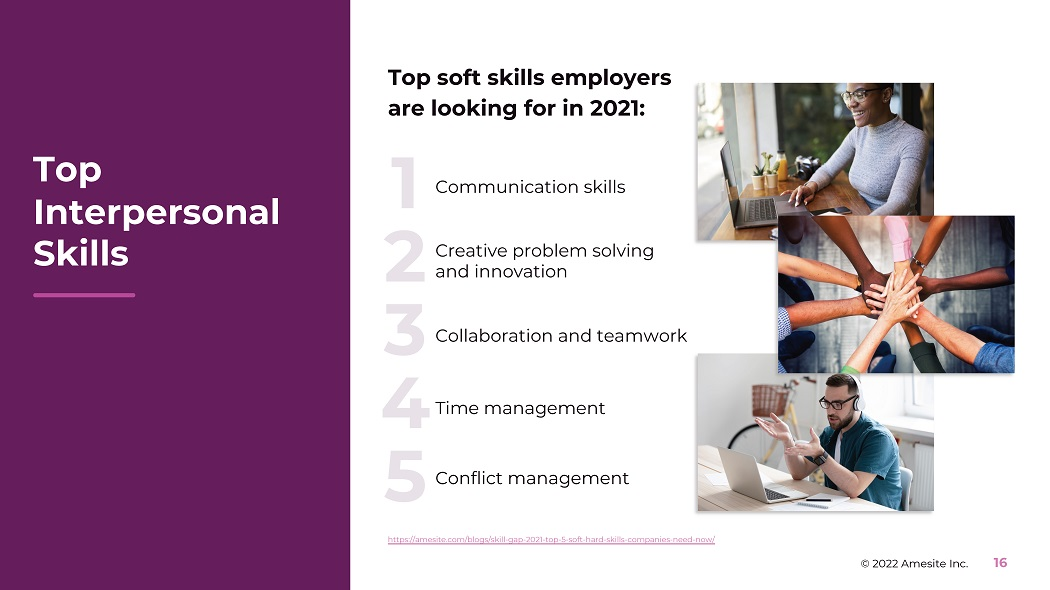Top soft skills employers are looking for in 2021: Co mm u n i c a t i o n s k ill s Cr e a t i v e p r o b l e m s o l v i n g https://amesite.com/blogs/skill - gap - 2021 - top - 5 - soft - hard - skills - companies - need - now/ © 2022 Amesite Inc. 16 a n d i nn o v a t i o n 1 2 3 Co ll a b o r a t i o n a n d t e a m w o r k 4 Ti m e m a n a g e m e n t 5 Co n f li c t m a n a g e m e n t Top In t e r p e r so nal Skills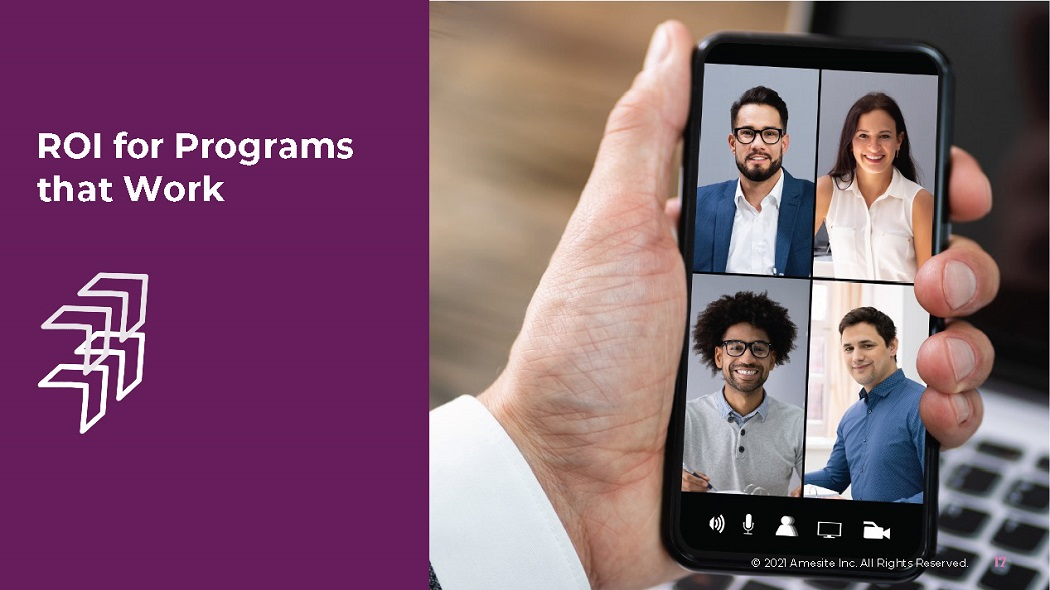ROI for Programs that Work © 2 0 2 1 A m e s i t e I nc . A l l R i g h t s R e s e r v e d . 17Up s k illi n g r e s u l t s i n a 300% i n c r e a s e i n d i g it al t r a n s f o r m a ti o n a cc e l e r a ti o n . Companies that Invest in Upskilling Report: 94% of e m p l o y ee s r e p o r t e d t h a t t h e y w o u l d s t a y l o n g e r a t a co m p a n y t h a t i n v e s t s i n t h e i r career. © 2022 Amesite Inc. 18 https://amesite.com/blogs/6 - myths - to - debunk - about - upskilling%e2%80%af/ Companies Win by UpskillingIncreasing Median Revenue by Upskilling the Workforce 24% m o r e p r o f i t f o r c o mp a n i e s t h a t i n v e s t \$ 1 , 5 00 o n t r a i n i n g p e r e m p l o y e e © 2022 Amesite Inc. 19 10% i n c r e a s e i n e d u c a t i o n al d e v e l o pm e n t p r o d u c e d a n 8.6% g a i n i n p r o d u c t i v i t y https://amesite.com/blogs/6 - myths - to - debunk - about - upskilling%e2%80%af/ https://amesite.com/blogs/why - your - business - cant - afford - not - to - upskill/ Upskilling ROI: By the NumbersK E Y R E Q U I R E M E N T S : SaaS learning solutions should not require expensive enterprise onboarding or training and should include CUSTOMER SERVICE and NO HIRING. © 2 0 2 1 A m e s i t e I nc . P r o p r i et a r y a n d C o n f i d e n t i a l . A l l R i g h t s R e s e r v e d . 20 Ease of Use is Essential for Learning Performance People Expect Ease of Use Ev e ry \$ 1 i n v e s t e d i n U X re s u l t s i n a re t u rn be t w ee n \$2 \$1 0 0 Int e n t i on a l a nd s t r a t e g i c u se r ex p eri en c e h a s t h e p o t e n t i a l t o r a i s e co n v e r s i o n r a t e s b y a s m uc h a s 40 0 % Sa a S c o mp a n y M c A fe e cu t t h e i r s u pp o r t c a ll s b y 90 % a s a r e s u l t o f u s e r i n t e r f ac e r e d e s i g n of c u s t o m e r s f ee l t h a t t h e c o m p a n i e s t h e y b u y fr o m ‘c o u l d d o be tt e r ’ w h e n i t c o m e s to onboarding new users. 9 0 % 8 0 % of u s e r s s a y t h e y ’ v e deleted an app be c a u s e t h e y d i dn ’ t know how to use it. 8 6 % of p e o p l e s a y t h e y ’ d be m o re li k e l y t o s t a y lo y a l t o a b u s i n e ss t h a t i n v e s t s in onboarding content. https://amesite.com/blogs/5 - simple - steps - to - deliver - online - learning - for - your - company/ https://amesite.com/blogs/how - to - attract - top - talent - with - your - workplace - education - benefits/ https://amesite.com/blogs/how - to - add - value - to - your - university / https://amesite.com/blogs/6 - myths - to - debunk - about - upskilling%e2%80%af / https://amesite.com/blogs/the - three - rs - retain - retrain - recruit - plus - the - secret - to - do - all - 3 - at - once/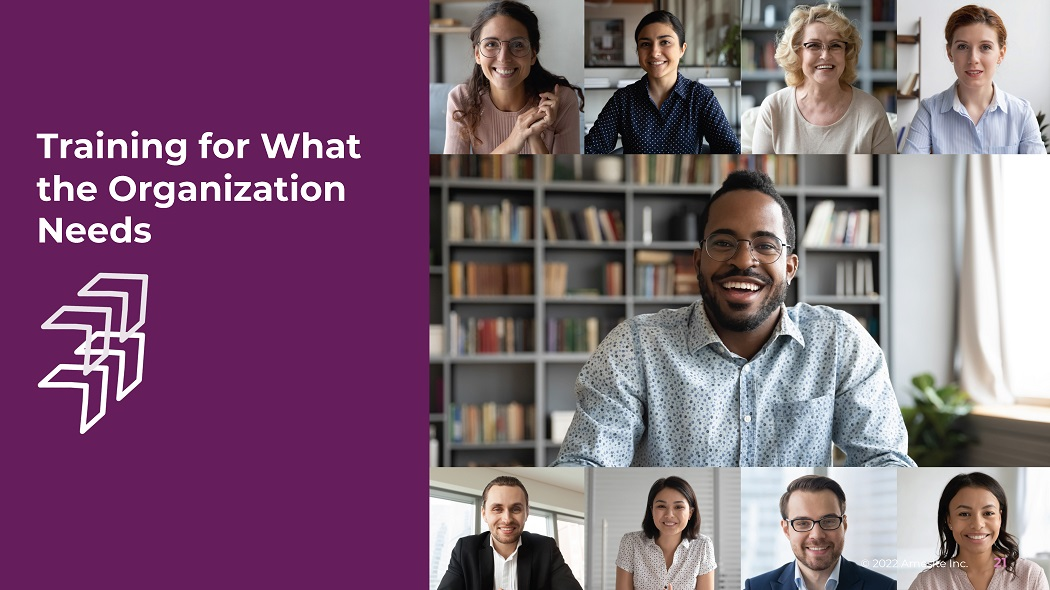Training for What the Organization Needs © 2022 Amesite Inc. 21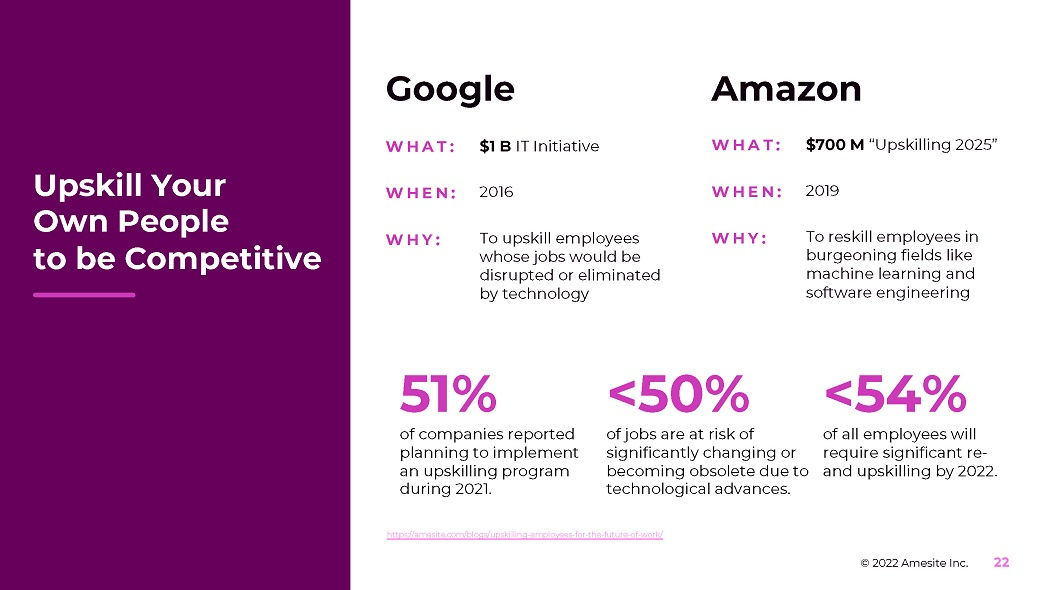Upskill Your Own People to be Competitive 51% of c o m p a n i e s r e p o r t e d p l a nn i n g t o i m p le m e n t a n u p s k illi n g p r o g r am d u r i n g 2 0 2 1 . <50% of j o b s a r e a t r i s k of s i g n i f i c a n t l y c h a n g i n g o r be c o m i n g o bs o l e t e d u e t o t e c hn o l o g i c a l a d v a n c e s . <54% o f a l l e m p l o y ee s w il l re q u ire s i g n i f i c a n t r e - a n d u p s k illi n g b y 2 0 22 . G o o g l e Amazon W H A T : \$7 0 0 M “U p s k illi ng 2 0 2 5 ” WH E N : 2019 W H A T : \$ 1 B IT In i t i a t i v e WH E N : 2016 W H Y : T o u p s ki l l e m p l o y ee s w h o s e j o b s w o u l d b e d i s ru p t e d o r e li m i n a t e d by t e c hn o l o g y W H Y : T o r e s ki l l e m p l o y ee s i n b u rg e o n i n g f i e l ds li ke m a c h i ne l e a r n i ng a nd s o f t w a r e e n g i n ee r i n g https://amesite.com/blogs/upskilling - employees - for - the - future - of - work/ © 2022 Amesite Inc. 22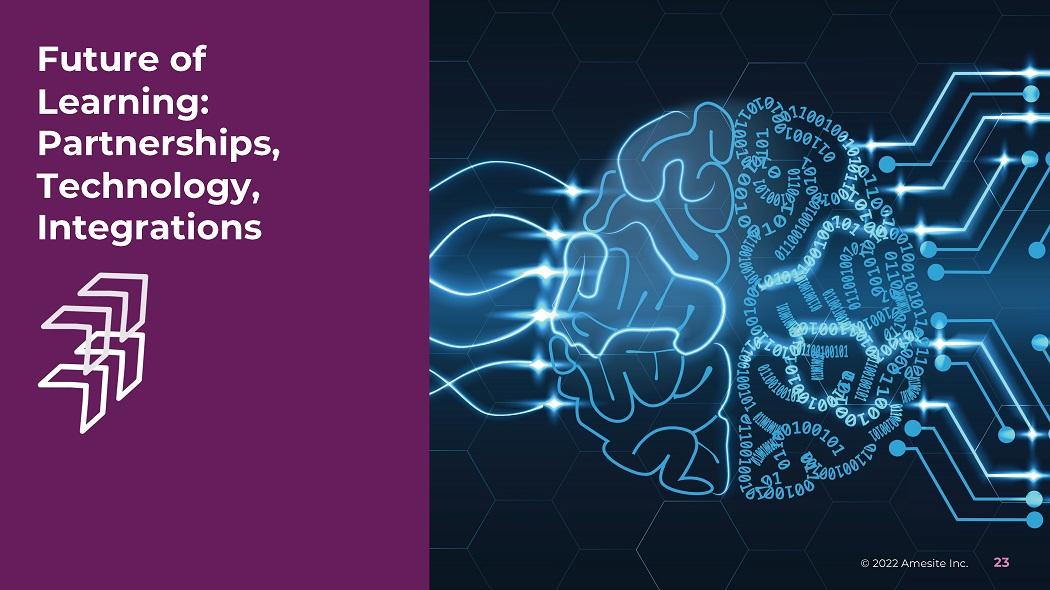Future of Learning: P a rt n e r s h i p s , Technology, Integrations © 2022 Amesite Inc. 23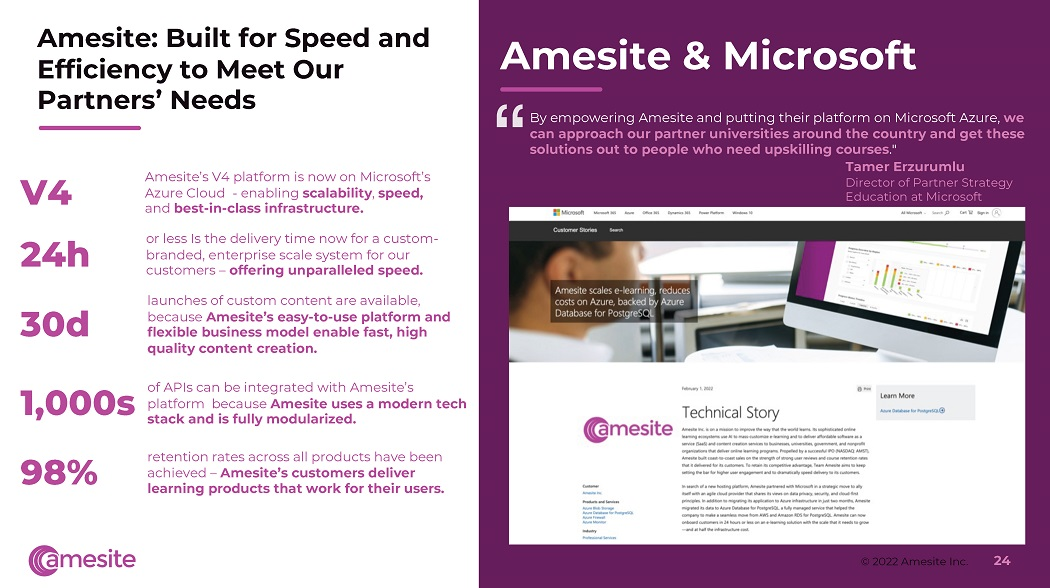A m e s i t e : B u il t f o r S p eed a n d E f f i ci e n c y t o M ee t O ur P a r t n e r s ’ N ee d s Amesite & Microsoft “ B y e m p o w e r i n g A m e s i te a n d p u tt i n g t h e i r p l a t f o r m o n M i c r o s o f t A z u r e , we can approach our partner universities around the country and get these solutions out to people who need upskilling courses . " Tamer Erzurumlu Di re c t o r o f P a r t n e r S t ra te g y Ed u c a t i o n a t M i c r o s o ft Am e s i t e ’ s V 4 p la t f o r m is n o w o n M i c r o s o f t ’ s Azure Cloud - enabling scalability , speed, an d be s t - i n - cl a ss i n f r a s t r u ctu r e . o r l e ss I s t h e d e li v e r y t i m e n o w f o r a c u s t o m - branded, enterprise scale system for our customers – offering unparalleled speed. la unch e s o f cu s t o m c o n te n t a r e a v a ila b l e , b e c a u s e A m e s i t e ’ s e a s y - to - use p l a t f o r m a n d flexible business model enable fast, high quality content creation. V4 24h 30d 1 , 000 s 98% of A P I s c a n b e i n te g r a te d w i t h A m e s i te ’ s p l a t f o r m b e c a u s e A m e s i t e u s e s a m o d e r n t e c h stack and is fully modularized. © 2022 Amesite Inc. 24 re t e n t i o n ra t e s a c r o ss a ll p r o d uc t s h a v e b ee n a c h i e v e d – A m e s i t e ’ s c u s t o m e r s d e l i v er learning products that work for their users.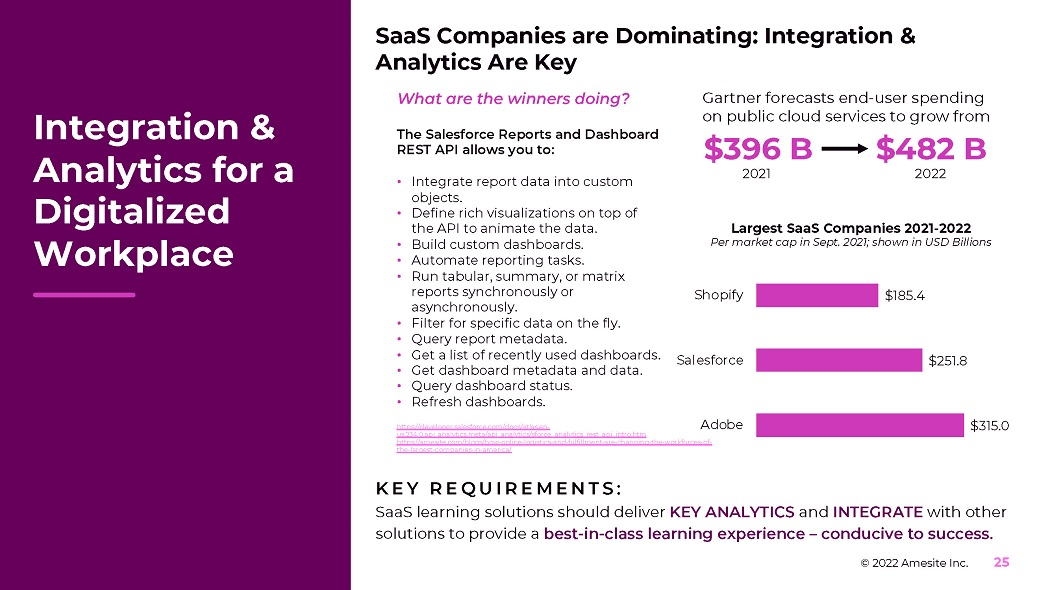SaaS Companies are Dominating: Integration & Analytics Are Key K E Y R E Q U I R E M E N T S : Sa a S l e a rn i n g s o l u t i o n s s h o u l d d e li v e r K E Y A N A L Y T I C S a n d IN T E G R A T E w i t h ot h e r solutions to provide a best - in - class learning experience – conducive to success. Integration & Analytics for a Digitalized Workplace G a r t n e r f o r e c a s t s e n d - u s e r s p e n d i ng on public cloud services to grow from \$396 B 2021 \$482 B 2022 \$31 5. 0 © 2022 Amesite Inc. 25 \$251.8 \$1 85. 4 A d o b e Salesforce Shopify Largest SaaS Companies 2021 - 2022 Per market cap in Sept. 2021; shown in USD Billions W h a t a r e t h e w i nn e r s d o i n g ? The Salesforce Reports and Dashboard REST API allows you to: • Integ r a te r e p o r t d a ta i nto cu s to m objects. • De f i n e r i c h v i s u a li z a t i o n s o n t o p o f the A P I to a ni m a te the d a t a . • Bu il d c u s t o m d a s h b o a r d s . • Au t o ma t e r e p o r t i n g t a s k s . • R u n t a b u l a r , s u mm a r y , o r m a t r i x re p o r t s s y nch r o n o u s l y o r asynchronously. • Fi l te r f o r s p e c i f i c d a t a o n t h e f l y . • Q u e r y r e p o r t m et a d a t a . • Ge t a li s t of r e c e n t l y u s e d d a s h b o a r d s . • Ge t d a s h b o a r d m e t a d a t a a n d d a t a . • Q u e r y d a s h b o a r d s t a t u s . • R e f r e s h d a s h b o a r d s . https://developer.salesforce.com/docs/atlas.en - us.234.0.api_analytics.meta/api_analytics/sforce_analytics_rest_api_intro.htm https://amesite.com/blogs/how - online - logistics - and - fulfillment - are - changing - the - workforces - of - the - largest - companies - in - america/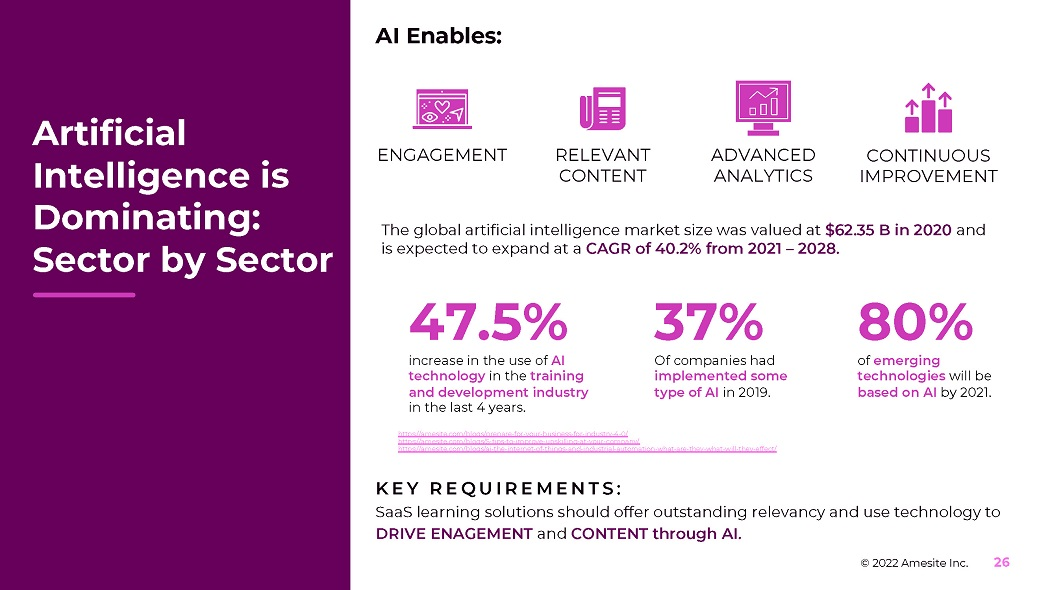K E Y R E Q U I R E M E N T S : SaaS learning solutions should offer outstanding relevancy and use technology to D R I V E E N A G E M E N T a n d C O N T E N T t h r o u g h A I . Artificial Intelligence is Dominating: Sector by Sector AI Enables: ENGAGEMENT R E L E V A N T CONTENT AD V AN C ED ANALYTICS CONTINUOUS I M P R O V E M E N T The global artificial intelligence market size was valued at \$62.35 B in 2020 and i s e x p e c t e d t o e x p a n d a t a C A G R o f 4 0 .2 % f r o m 2 0 2 1 – 20 28 . © 2022 Amesite Inc. 26 47.5% i n c r ea s e i n the u s e o f AI tec hn o lo g y in t h e t r a inin g and development industry in t h e la s t 4 y e a r s . https://amesite.com/blogs/prepare - for - your - business - for - industry - 4 - 0 / 37% O f c o m p a n i e s h a d i m p le m ented s o m e t y p e o f A I in 2 0 1 9 . 80% of e m e r g in g tec hn o lo g ies w i ll b e b a s e d o n A I b y 2 0 2 1 . https://amesite.com/blogs/5 - tips - to - improve - upskilling - at - your - company/ https://amesite.com/blogs/ai - the - internet - of - things - and - industrial - automation - what - are - they - what - will - they - effect/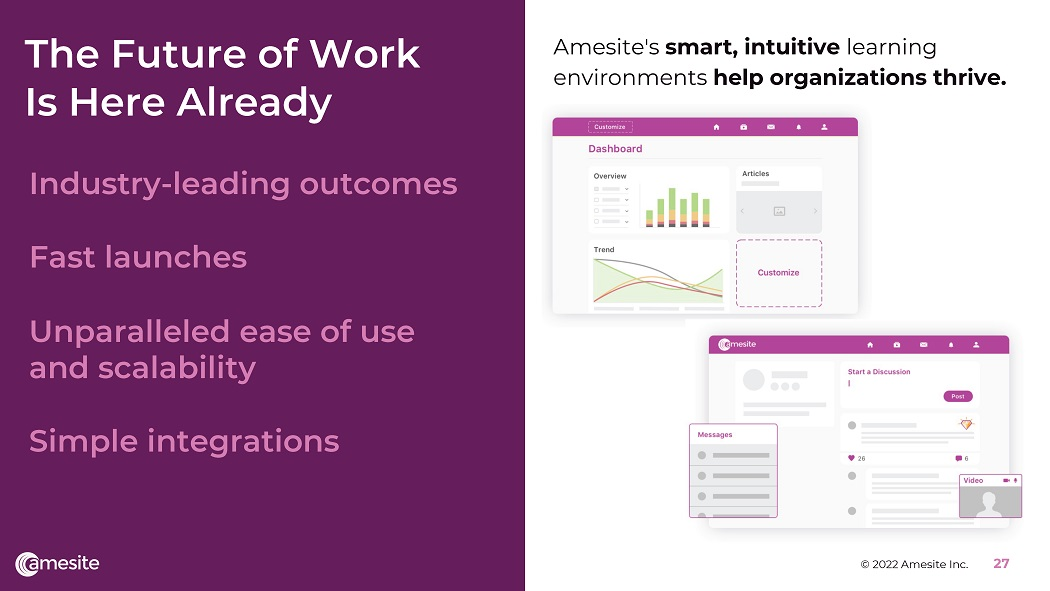The Future of Work Is H e re A l r e ady Industry - leading outcomes Fast launches Unparalleled ease of use and scalability Simple integrations Ame s i t e ' s sm a rt , i n t u i t i v e l e a r n in g e n v i r o n m e n t s h e l p o r ga n i z a t i o n s t h r i v e . © 2022 Amesite Inc. 27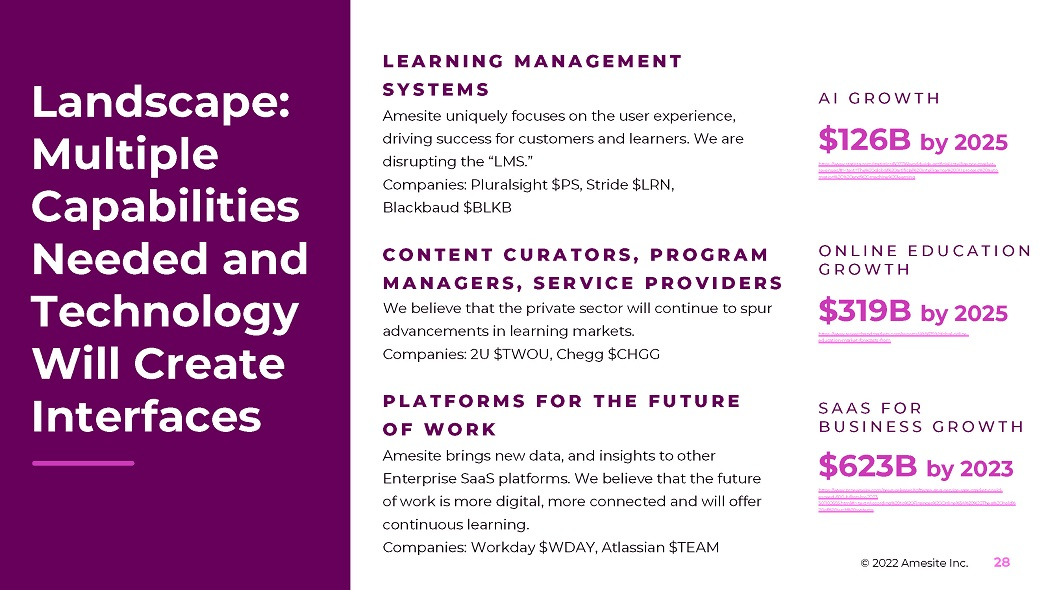Landscape: Multiple Capabilities Needed and Technology Will Create Interfaces L E A R N I N G M A N A G E M E N T S Y S T E M S A m e s i te un i q u e l y f o c u s e s o n t h e u s e r e xp e r i e n c e , d r i v i n g s u cce ss f o r cu s t o m e r s a n d l e a r n e r s . W e a r e d i s r u p t i n g t h e “ L M S . ” C o m p a n i es : P l u r a l s i g h t \$ P S , S t r i d e \$ L R N , B l a c k b a u d \$ B L K B C O N T E N T C U R A T O R S , P R O G R A M M A N A G E R S , S E R V I C E P R O V I D E R S We believe that the private sector will continue to spur a d van ce m e nt s i n l e a r n i ng ma r k et s . C o m p a n i es : 2 U \$ T W O U , C h e g g \$ C H G G P L A T F O R M S F O R T H E F U T U R E O F W O R K A m e s i te b r i n g s n e w d a t a , a n d i n s i g h t s t o o t h e r Enterprise SaaS platforms. We believe that the future of w o r k i s m o r e d i g i t al , m o r e c o n n e c t e d a n d w ill of f e r c o n t i nu o u s l e a r n i n g . C o m p a n i es : W o r k d a y \$ W D A Y , A t l a ss i a n \$ T E A M A I G R O W T H \$126B by 2025 https:// www.statista.com/statistics/607716/worldwide - artificial - intelligence - market - revenues/#:~:text=The%20global%20artificial%20intelligence%20(AI,process%20auto mation%2C%20and%20machine%20learning . O N L I N E E D U C A T I O N GR O W T H \$319B by 2025 https:// www.researchandmarkets.com/reports/4986759/global - online - education - market - forecasts - from S A A S F O R B U S I N E S S G R O W T H \$623B by 2023 https:// www.prnewswire.com/news - releases/software - as - a - service - saas - market - could - exceed - 600 - billion - by - 2023 © 2022 Amesite Inc. 28 301102655.html#:~:text=According%20to%20Finances%20Online%3A%20%22The,a%20hold% 20of%20such%20system s.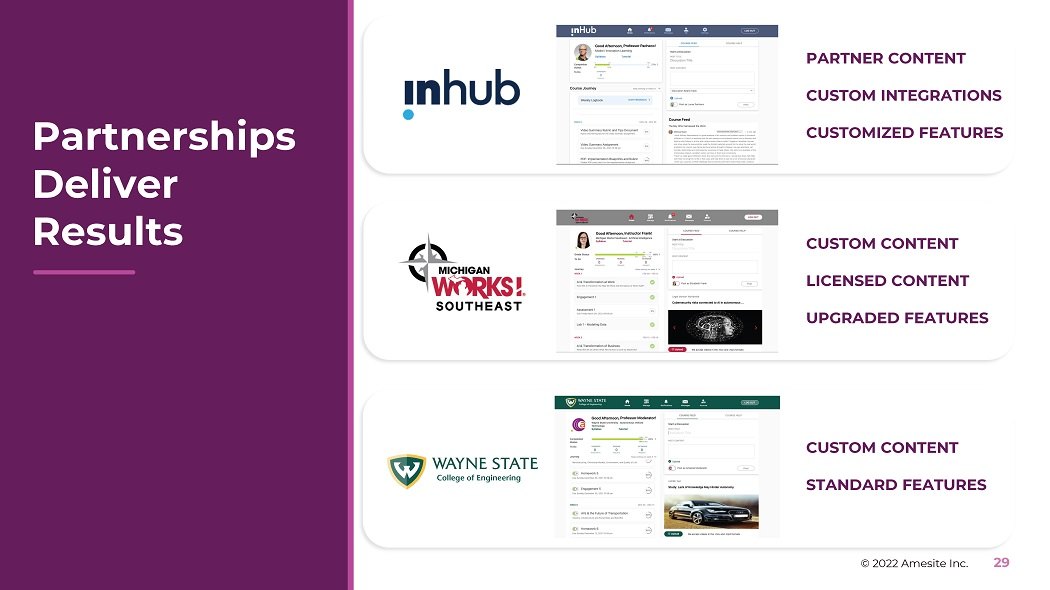P a r t ne r s hi p s Deliver Results PARTNER CONTENT CUSTOM INTEGRATIONS CUSTOMIZED FEATURES CUSTOM CONTENT LICENSED CONTENT UPGRADED FEATURES CUSTOM CONTENT STANDARD FEATURES © 2022 Amesite Inc. 29P R O DU C T D E M O N S T R A T I O N S © 2022 Amesite Inc. 30 EB O O K S P R E S E N T A T I O N S brandon@amesite.io FOR MORE IN F O R M A T IONSL I D E T I T LE © 2 0 2 1 A m e s i t e I nc . A l l R i g h t s R e s e r v e d . P r o p r i et a r y & C o n f i d e n t i a l . 31 T H A N K Y OU . F O L L O W O U R P RO G RE S S F O R R ES O U R C E S F O R I N V ES T M E N T